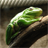# extracting values by using dictionary key

2548
11
09-23-2014 09:51 AMNew Contributor

Hi Guys,

I have text file having two columns: 1) column 1 is in the format yyyy-mm-dd and 2) column 2 is precipitation.

Objective: to extract original value of precipitation from column 2 only for month April, May, june, July, and August (4,5,6,7,8).

Procedure: Used line.split to extract only mm from yyyy-mm-dd format from column-1.

• made dictionary to get month and precipitation value.
• using for k,v in dct.items() and then using a if statement to extract the corresponding month precipitation value from dictionary

Problem: I can successfully print k,v (month and precipitation) from dictionary items. However, when I am using a if statement to extract the specific month's precipitation value, I am getting blank array. I was wondering can I use .append to get precipitation in `(1.8,2.1,3.3)` format.

Code:

`file1 = open("test.txt","r")  Growing=[] Intermediate=[] Dormant=[]  for line in file1:     line2 = line.split()     WQ = line2     month = line2.split("-")     dct1={month:WQ}     for k,v in dct1.items():         if (k ==4 or k==5 or k==6 or k==7 or k==8):             Growing.append(dct1 )     print Growing`
Tags (3)
1 Solution

Accepted SolutionsbyEsri Esteemed Contributor

Using the attached sample file and the code below I obtained a list of precipitation values:

```infile = r"D:\Xander\txt\precip.txt"
sep = "\t" # example TAB as seperator

lst_months = [4, 5, 6, 7, 8]
growing = []
i = 0
with open(infile,"r") as f:
for line in f:
i += 1
line = line.strip("\n")
data = line.split(sep)
if len(data) == 2:
print data
date = data
prec = float(data)
month = int(date.split("-"))
if month in lst_months:
growing.append(prec)

print growing
```

Kind regards, Xander

11 RepliesbyEsri Esteemed Contributor

Using the attached sample file and the code below I obtained a list of precipitation values:

```infile = r"D:\Xander\txt\precip.txt"
sep = "\t" # example TAB as seperator

lst_months = [4, 5, 6, 7, 8]
growing = []
i = 0
with open(infile,"r") as f:
for line in f:
i += 1
line = line.strip("\n")
data = line.split(sep)
if len(data) == 2:
print data
date = data
prec = float(data)
month = int(date.split("-"))
if month in lst_months:
growing.append(prec)

print growing
```

Kind regards, XanderNew Contributor

Hi Xander,

Thanks a lot for your help! I was stuck and it really helped me.Yeah, the code is working perfect. Appreciate it. Have a good one!

SeanbyEsri Esteemed Contributor

Hi Sean, you´re welcome. Can you mark the question as answered?

Kind regards, XanderNew Contributor

How do I mark as answered. I looked at and found a link called as mark as helpful and did that. Is that what you wanted me to do?byEsri Esteemed Contributor

There should be a star "Correct Answer" that you can press next to the post that answered you question.New Contributor

I think I marked as answer  now. Thank you!byEsri Esteemed Contributor

Yes you did. Thanks!New Contributor

Hi Xander,

Just a silly question. I want to extract the corresponding date of the precip along with the precip value. For that, I added one line code: growing.append(float(date)) after growing.append(prec) in your code.

However, I got this error:"ValueError: invalid literal for float(): 2007-05-07". Do you mind to look at it?

Thank you very much for your time and help!

SeanbyEsri Esteemed Contributor

Hi Sean,

Basically the error is telling you that the date (2007-05-07) is not a float (decimal value). The other thing is that your code will result in a list that holds a precipitation value, a date, a precipitation value, a date, etc. It would probably be more appropriate to work with a nested list like this:

```date = data
prec = float(data)
lst_date_prec = [date, prec]
month = int(date.split("-"))
if month in lst_months:
growing.append(lst_date_prec)
```

This results i a list where each item contains a list of a precipitation value and the corresponding date. It really depends on what you want to do with the list...

Kind regards, Xander